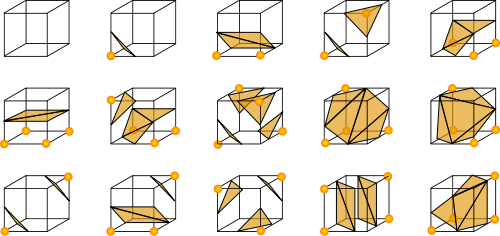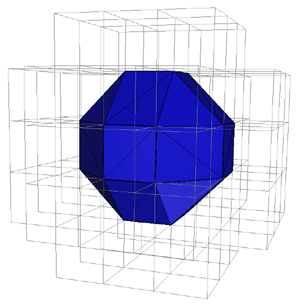# Animating Isosurfaces with WebGL and Workers

Posted in: javascript , webgl

If you just want to take a look at the JavaScript demos with WebGL and Workers you can click here or just go towards the bottom of the page

For some time now I’ve been considering rendering isosurfaces with JavaScript. Isosurfaces are 3D surfaces that can be defined with implicit 3D equations.

While “regular” 3D surfaces are defined as:

``z = f(x, y);``

isosurfaces can also be represented as:

``c = f(x, y, z);``

Some shapes that can be represented as implicit equations and that can’t be rendered just as “regular” 3D surfaces include the Sphere. While with regular surfaces we can have either the top or bottom part of a Sphere:

``````z = f(x, y); // ->

z = (+|-)Math.sqrt(1 - x * x - y * y); //either + or -``````

Implicit 3D equations can define the whole shape:

``````c = f(x, y, z); // ->

1 = x * x + y * y + z * z;``````

Implicit 3D equations can be used to model organs after taking a computed tomography, or to model other physical phenomena (like fluids), or even to do old-style OpenGL animated demos like Metaballs :P

## How do I render an Isosurface?

There’s this very interesting Marching Cubes algorithm that describes how to create primitives that can be later sent to an OpenGL renderer in order to plot Isosurfaces (the primitives are triangles in this case). The main principle of the algorithm is to create some sort of differentials by dividing the space in which the surface is defined into “very small” cubes within the main volume where the visualization is contained and study how the surface intersects these small cubes (when it does). We can identify in this way 256 different cases in which the surface can “cut” these small cubes:For each of these cases we can obtain the group of triangles corresponding to the part of the surface that intersected the small cube. That way we can recreate the surface to be rendered.## Approaching the Problem with WebGL

The power of WebGL lies in being able to compute these algorithms in some shader language. In the WebGL programable pipeline we can write shader code at two different stages: when processing a vertex (vertex shader) or when doing per pixel manipulation (fragment shader). Unfortunately this means that we are forced to send to the vertex shader an array of predefined vertices to render the surface before computing anything on the GPU. There is no way to send any other type of information through the shader that once arrived to the vertex shader would allow it to “emit” new vertices.

For example, one approach I would have enjoyed doing is to send the grid details to the shaders and then calculate the triangles and emit new vertices to render the scene from the shader. However I haven’t found a way to do this with WebGL because there’s no geometry shader.

So we’re stuck on the client-side to calculate all vertices and then send the information to the vertex shader with WebGL. This proves to be a little bit slow.

## Web Workers to the rescue!

The divide & conquer nature of the algorithm made me think that I could divide the computing space into an octree and parallelize each octant with a Worker. A worker is a JavaScript API that provides asynchronous threads of execution. One can post messages to the workers and add onmessage listeners to them to do something in particular when they finish the computation or they send us some message. Workers can call other workers and also one can create multiple workers out of the same code. For more information about Workers read the link above ;).

So now what I needed is something like a WorkerGroup that would create a certain number of workers and map different configurations for each of them, and then reduce all workers responses into a single “merged” result of vertices to send to the shaders.

A way to implement the WorkerGroup would be:

``````//Create an array of workers
function WorkerGroup(fileName, n) {
var workers = this.workers = [];
while (n--) {
workers.push(new Worker(fileName));
}
}

WorkerGroup.prototype = {
//create a configuration array to post to each worker
//when reduce is called
map: function(callback) {
var workers = this.workers;
var configs = this.configs = [];

for (var i = 0, l = workers.length; i < l; i++) {
configs.push(callback(i));
}
},
//run all workers with their proper configuration and
//define a group-callback to merge the results
reduce: function(opt) {
var fn = opt.reduceFn,
workers = this.workers,
configs = this.configs,
l = workers.length,
acum = opt.initialValue,
message = function (e) {
l--;
if (acum === undefined) {
acum = e.data;
} else {
acum = fn(acum, e.data);
}
if (l == 0) {
opt.onComplete(acum);
}
};
for (var i = 0, ln = l; i < ln; i++) {
var w = workers[i];
w.onmessage = message;
w.postMessage(configs[i]);
}
}
};``````

The performance improvements of using Workers are very noticeable. The algorithm remains on the client-side though, so if you want fast performance I’d recommend you to use some webkit nightly.

## The Result

I made two demos, one is a Metaballs demo and the other one is an Isosurface visualizer. I really like the second example because you can specify your own formulas. There are predefined formulas like a Torus or Gyroid. To see these demos you’ll need a WebGL enabled browser, I do encourage you to install a webkit nightly for this.

Click here to take a look at the Metaballs demo, or you can watch a video here:

For the Isosurface generator you can click here or take a look at the video below:

## GitHub

I’m pushing all experiments from now on to a Playground repo for you to check the code or improve. There’s also some 2D Canvas, WebGL and Scala code from Three ways to make 3D there.